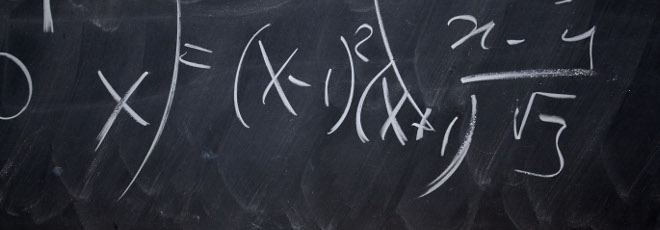## “On semi-classical spectral series for an atom in a periodic circularly polarized electric field”

Venerdì 16 Luglio 2021, ore 10:30 - Zoom - Michel Rouleux (CPT - Université de Toulon)

Abstract

We consider time-dependent Schroedinger operators of type $H(t)=-h^2 \Delta+V(x)+E(t)\cdot x$ where $V$ is a radial potential and $E(t)=(\cos{(\omega t)}, \sin{(\omega t)}, 0)$ is a circularly polarized field. Monodromy operator $U(T,0)$ that takes the system through a complete period $T=2\pi/\omega$, is unitarily equivalent to $e^{iTP(x,hD_x)/h}$, where $P(x,hD_x)$ is a magnetic Schroedinger operator. If $V(r)$ has a non degenerate critical point at $r_0$ then, for some values of the parameters, the Hamiltonian $p(x,\xi)$ has elliptic fixed points $\rho_0^\pm=(x_0,\xi_0)_\pm$ in the phase space. We construct quasi-modes supported microlocally near $\rho_0^\pm$. Moreover, when $V$ is a confining potential at infinity, we examine tunneling between these points and we estimate the splitting between the two first eigenvalues in the semi-classical limit.